# ASVAB Mechanical Comprehension Practice Test

This is our free ASVAB Mechanical Comprehension practice test. On the Mechanical Comprehension ASVAB test you will be given 20 minutes in which to complete 16 questions. You will need to be familiar with mechanics and applied physics. Topics will include basic mechanics, simple machines and fluid power. If you spend some time reviewing this material it will make a big difference in your final score. Start your review now with our ASVAB Mechanical Comprehension practice questions.

Congratulations - you have completed . You scored %%SCORE%% out of %%TOTAL%%. Your performance has been rated as %%RATING%%
 Question 1

### Which of the following is the basis for Pascal’s Law?

 A The amplification of force in a hydraulic system. B The relationship between force and volume of a liquid. C The definition of pressure as pounds per square inch. D The manner in which liquids conform to their container.
Question 1 Explanation:
The correct answer is (A). The third principle of hydraulics is the basis for Pascal’s Law and states that when pressure is applied to a fluid, the pressure is transmitted evenly throughout—in other words, how force is amplified within a hydraulic system.
 Question 2

### The smaller gear has 8 teeth, and the larger gear has 16 teeth. When the larger gear makes 12 revolutions, how many revolutions will the smaller gear make?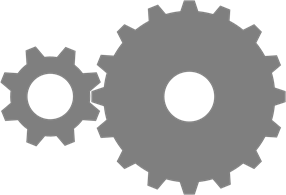A 8 B 16 C 18 D 24
Question 2 Explanation:
The correct answer is (D). The gear ratio here is 2:1, meaning that for every revolution of the larger gear, the smaller gear revolves twice. If the larger gear revolves 12 times, the smaller gear will revolve twice as many times.
 Question 3

### How much force is needed to lift the 10Kg weight?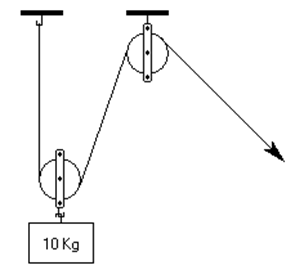A 2 Kg B 5 Kg C 10 Kg D 20 Kg
Question 3 Explanation:
The correct answer is (B). In a double-pulley system, the force is equal to the weight divided by two. It will require a 5 Kg force to lift a 10Kg weight.
 Question 4

### An 80-lb child is sitting on one end of a seesaw. If another 80-lb child jumps from a high platform onto the other end of the seesaw, what will happen?

 A The first child will stay stationary on the ground. B The second child will bounce off the seesaw into the air. C The first child will bounce off the seesaw into the air. D The first child will be lifted into the air, but remain seated.
Question 4 Explanation:
The correct answer is (D). The force of the second child landing on the seesaw will propel the other end of the seesaw into the air.
 Question 5

### What is a vector quantity?

 A Gravity B Magnitude C Volume D Mass
Question 5 Explanation:
The correct answer is (A). Gravity is a type of force, and since force has magnitude and direction it is known as a vector quantity.
 Question 6

### Which of the following describes the relationship between kinetic energy, mass, and velocity?

 A KE = ½ m2v B (1/3)(KE) = m/v2 C 2(KE) = mv2 D 3(KE) = m2v
Question 6 Explanation:
The correct answer is (C). An object’s kinetic energy is defined as KE = ½(mv2). Reorienting, we find: 2KE = mv2.
 Question 7

### One horsepower is equivalent to

 A 724 watts B 746 watts C 768 watts D 782 watts
Question 7 Explanation:
The correct answer is (B). Horsepower is a unit used to rate internal combustion engines. One horsepower is the equivalent of 746 watts.
 Question 8

### What type of simple machine is pictured below?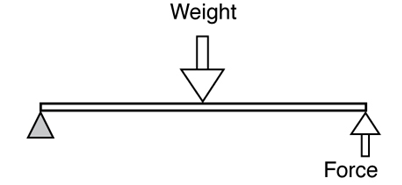A Fulcrum B First-class lever C Second-class lever D Third-class lever
Question 8 Explanation:
The correct answer is (C). This machine pictured is a second-class lever. An example of a second-class lever is a wheelbarrow.
 Question 9

### At minimum, how many pulleys are there in a block and tackle system?

 A One B Two C Three D Four
Question 9 Explanation:
The correct answer is (B). The block and tackle pulley system requires at least two or more pulleys. The bare minimum for this system is two.
 Question 10

### When torque increases, speed

 A remains the same B increases, then decreases C increases D decreases
Question 10 Explanation:
The correct answer is (D). The quantities of torque and speed are inversely proportional. When one increases, the other decreases.
 Question 11

### If a wedge is made longer relative to its height, how does the force increase?

 A The amount of lift is decreased as the wedge is moved horizontally. B The amount of lift is increased as the wedge is moved horizontally. C The amount of lift is decreased as the wedge is moved vertically. D The amount of lift is increased as the wedge is moved vertically.
Question 11 Explanation:
The correct answer is (A). When the distance of a lift is the same as the distance the initial force travels, the force in will equal the force out. Making the wedge longer relative to its height (less slope) will change this by decreasing the amount of lift that takes place as the wedge is moved horizontally.
 Question 12

### If a man uses 200 N of force over a distance of 50 meters, how many joules of work are performed?

 A 1,000 B 5,000 C 10,000 D 20,000
Question 12 Explanation:
The correct answer is (C). Work is defined as the product of force in the direction of displacement. Here a force of 200 N is used over a displacement of 50 m. Consequently, the work performed is 200 N * 50 m = 10,000 J.
 Question 13

### What is a component of potential energy?

 A Upward reactive force B The energy of movement C Gravity’s relationship to mass D Newtons and distance
Question 13 Explanation:
The correct answer is (C). An object’s potential energy is equal to its mass times its acceleration due to gravity times its height, or PE = mgh.
 Question 14

### A fish tank at an aquarium contains several cubic feet of water (1 cubic foot of water = 62.5 pounds). If the fish tank is 6 feet deep, 12 feet wide, and 13 feet long, what’s the approximate pressure at the bottom of the tank in pounds per square inch?

 A 2 B 2.5 C 3 D 3.5
Question 14 Explanation:
The correct answer is (B). To find the pressure (psi), we must divide the tank’s total volume by the surface area of the bottom of the tank. The volume = 6 × 12 × 13 = 936 feet. Multiply it by 62.5 to convert it into pounds: 936 × 62.5 = 58,500.

The bottom of the tank’s surface area is 12 ft × 13 ft = 144 inches × 156 inches. Since pressure is in pounds per square inch (psi), we have to convert to inches. The surface area is 22,464 inches.

The psi is 58,500 ÷ 22,464 which is approximately 2.6. The closest answer is (B).

 Question 15

### If Gear A turns counterclockwise, what other gears turn counterclockwise?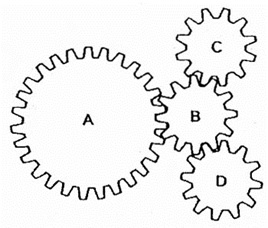A C and D B B and D C C and B D B, C, and D
Question 15 Explanation:
The correct answer is (A). If A turns counterclockwise, it will cause B to turn clockwise. B will cause C and D to turn counterclockwise.
 Question 16

### The well pictured below uses what type of simple machine to raise and lower a bucket?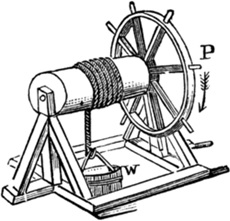A Block and tackle B Third-class lever C Wedge D Wheel and axle
Question 16 Explanation:
The correct answer is (D). The well pictured here uses a wheel and axle system. The movement at the wheel becomes smaller than the movement on the shaft, increasing the force on the shaft.
Once you are finished, click the button below. Any items you have not completed will be marked incorrect.
There are 16 questions to complete.
 ← List →